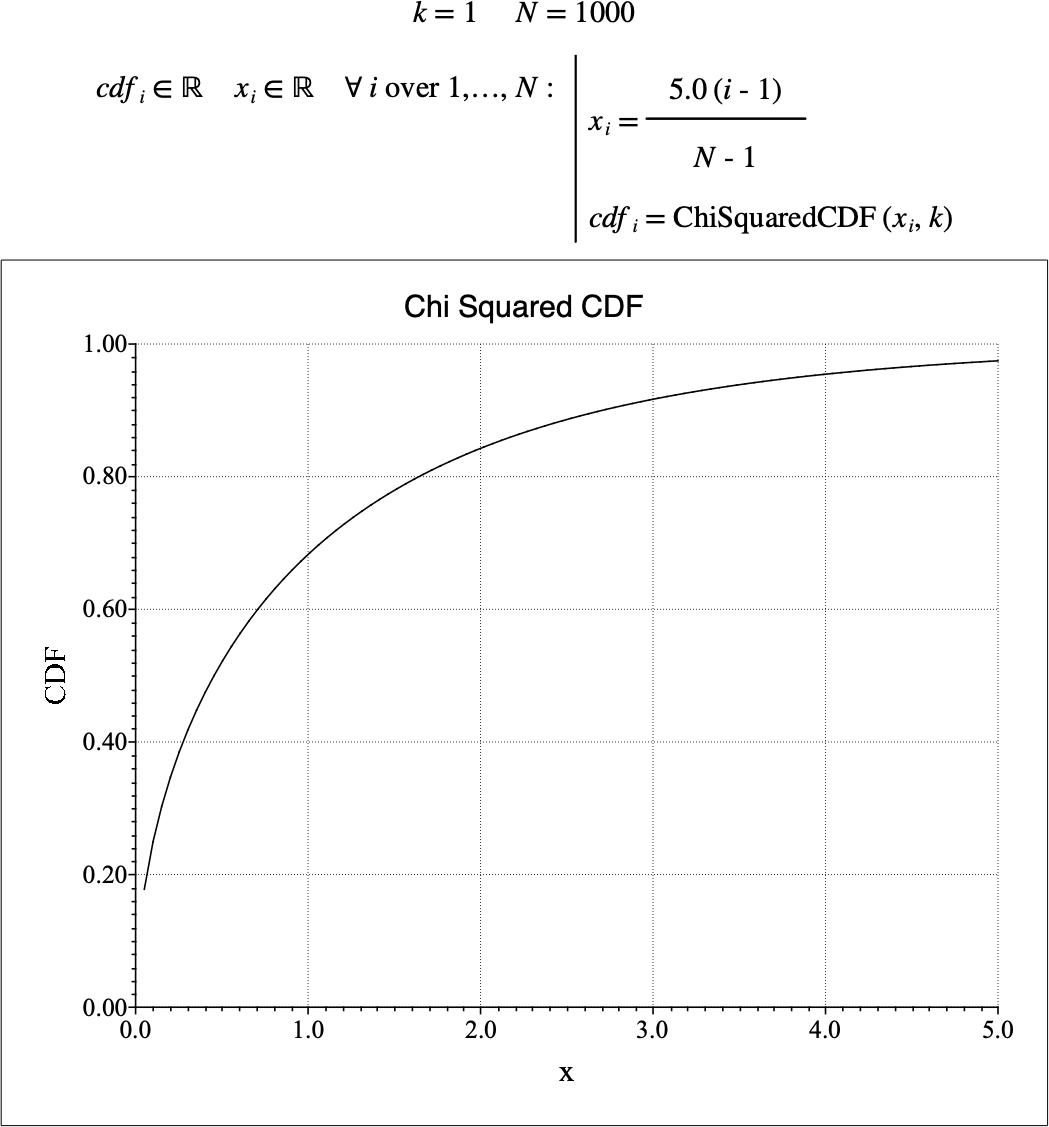# $$\text{ChiSquaredCDF}$$¶

You can use the $$\text{ChiSquaredCDF}$$ function to calculate the cumulative distribution function (CDF) of the chi-squared distribution.

You can use the \chisquaredc backslash command to insert this function.

The following variants of this function are available:

• $$\text{real } \text{ChiSquaredCDF} \left ( \text{<x>}, \text{<k>} \right )$$

Where $$x$$ and $$k$$ are scalar values indicating the value of interest and the degrees of freedom respectively. The function is defined for all $$k \in \mathbb{Z}$$ where $$k > 0$$ and where $$0 < x$$ if $$k = 1$$ and $$0 \leq x$$ for all other values of $$k$$.

The value is calculated directly using the relation:

$\text{ChiSquaredCDF} \left ( x, k \right ) = \text{P} \left ( \frac{k}{2}, \frac{x}{2} \right )$

Where $$\text{P}$$ is the regularized lower gamma function defined as:

$\text{P} \left ( s, z \right ) = \frac{\gamma \left ( s, x \right )}{\Gamma \left ( s \right )}$

The chi-squared distribution represents the sum of the squares of $$k$$ standard normally distributed random variates.

Figure 116 shows the basic use of the $$\text{ChiSquaredCDF}$$ function.Figure 116 Example Use Of the ChiSquaredCDF Function